LASCO and EIT Introduction Summary of LASCO/EIT What is LASCO? What is EIT? (external link) FAQ Real Time Data Products Realtime Images RealTime Movies (SOHO Movie Theater) Archive Data Products Database Queries and Download CME Queries (New!) Data Products Image Gallery Movie Gallery Processing Levels FITS Header Keywords Data Policy Wavelet images/movies Science Information Coronal Mass Ejections LASCO CDROMs Eclipse Observations Carrington Maps LASCO Calibration LASCO Sky Map LASCO C3 Planet transits (via Sungrazer) Latest Site Updates Technical Team and Operations Resources LASCO/C1 at MPAe (Germany) LASCO at LAS (France) LASCO Handbook Technical Notes Detailed Documentation Acronyms Related Links Solwind Images and CMEs SOHO Home page SOHO and SOHO Instruments Other Solar Satellites and Observatories LASCO C3 Stars and Vignetting Please direct comments or questions about this page to the webmaster . Last modified 22 Nov 2000. 1. Plot of all star trails with relative intensity (not normalized) for January 1998. To find the star brightness, I first subtract a point-filtered version of the image from that image, leaving only stars and cosmic rays (mostly). I assume the star is located at the pixel where the star has the brightest intensity--I also use this pixel to find the star. I then take the brightness of the star to be the sum of all the values in a 5x5 pixel box centered on the brightest pixel. 2. Plot of all of the star trails used for my calculation, normalized so the "regular" (unvignetted) value is 1. There are 257 star trails taken from June, October and December of 1996 and 1997 and January of 1997 and 1998. Color table 12 is applied, and the values are scaled between 0 and 1.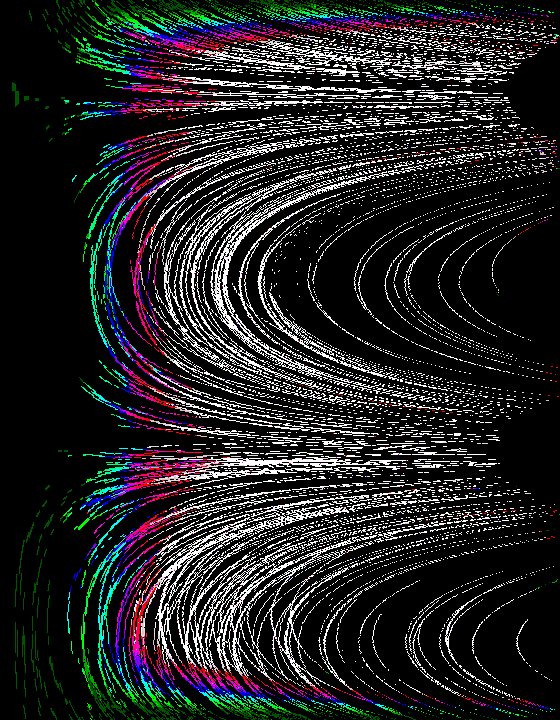3. Star trails from 4 ~15 day periods in 1996, 1997 and 1998 mapped into r-theta coordinates. In this figure, the x-axis is equivalent to r (1 pixel = 1 pixel), and the y-axis is equivalent to theta (1 pixel = 0.5 degrees).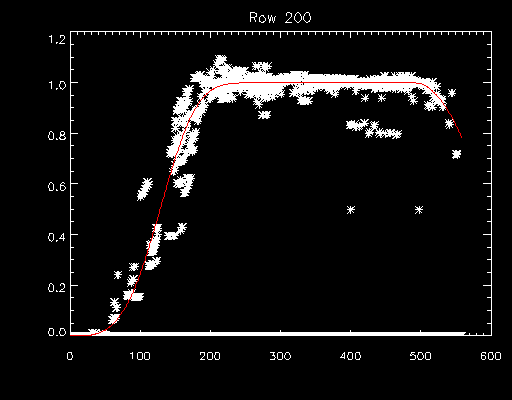4. Plot of star values from row 200 (approximately SW, in lower right quadrant) +/- 5 rows with computed value for row 200 in red. The the values for 0 to 480 are a fit to a function of the form y = 1 - exp(A*x^B). The values used to start out were A=-1e-7 and B=3. For computing the fit, 3 rows were used, instead of the five shown here.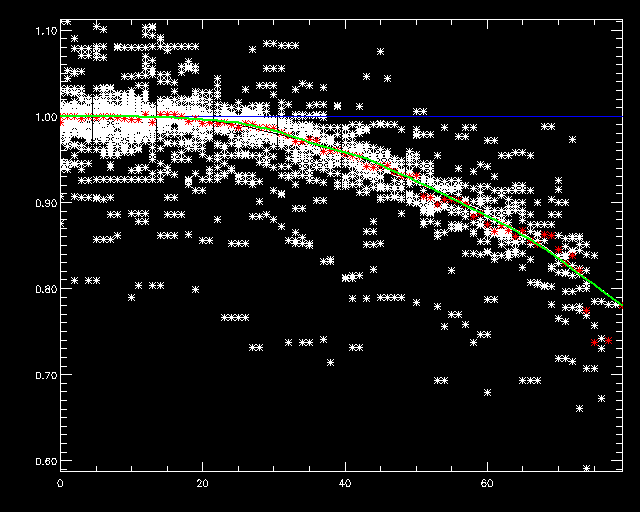5. This is a plot of star values for the outer edge (480 to 559 pixels from center of occulter) of the FOV over roughly 90 to 360 degrees (no pylon). The red dots are the mean for each r (or x) value. The green line is the exponential fit y = 1 + A - A*exp((B*x)^C) where A=0.13256092, B=0.088888151, C=0.65249860. The outer corners were done manually (see below).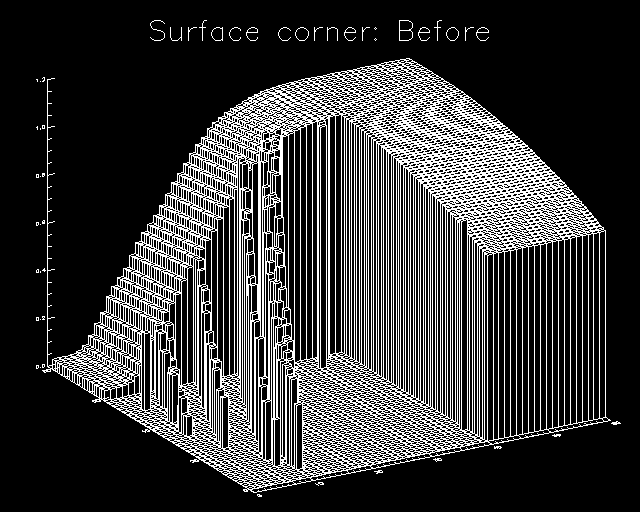6. This is what the outer corner of the vignetting surface fit looked like before extrapolating. (Equivalent to lower right corner of figure 3 above.)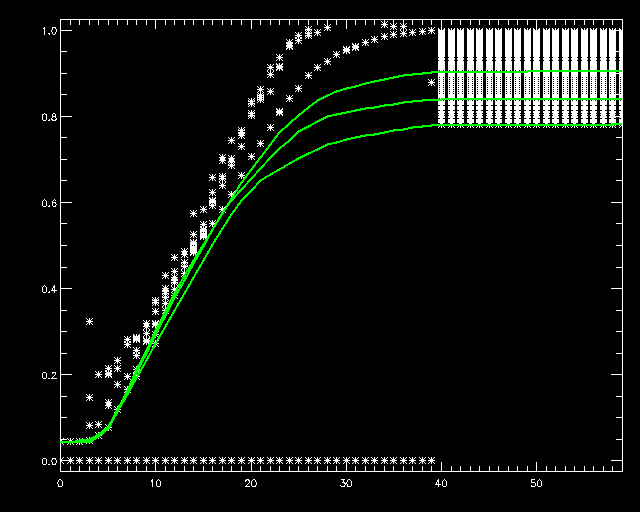7. I created curves by hand to cover the outer corners and then interpolated.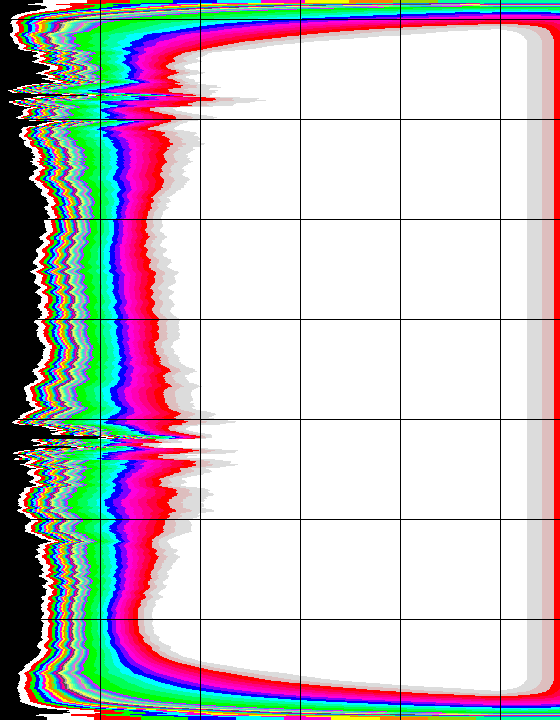8. Here is the result of doing all rows as described in #4 above. The black lines mark 100 pixels. the discontinuities near y = 280 and 620 correspond to rays parallel to star motion in the field of view.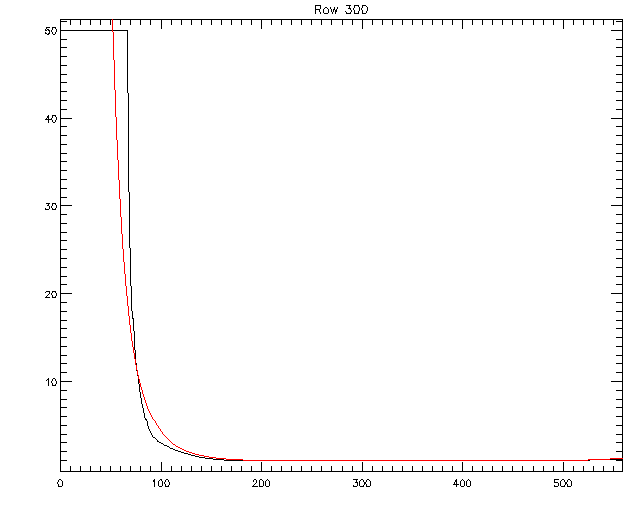9. Here is a plot of a ray of the August 1998 vignetting correction (black), and the fit computed from star values (red). Row 300 is about 20 degrees N of due W.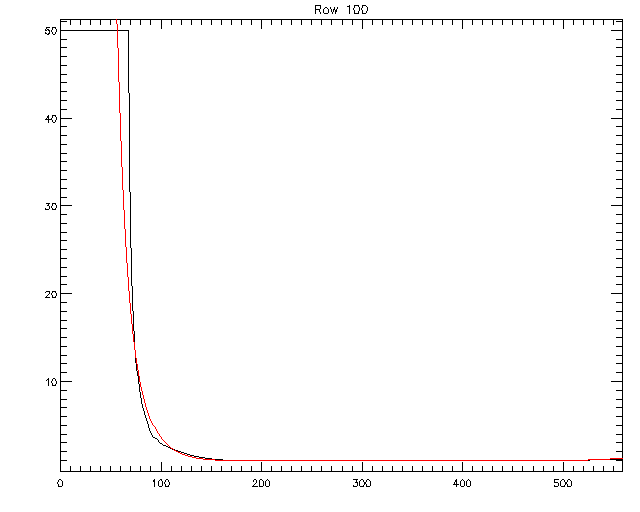10. Here is a plot of row 100, about 90 degrees (S pole). Black is old value, red is new values.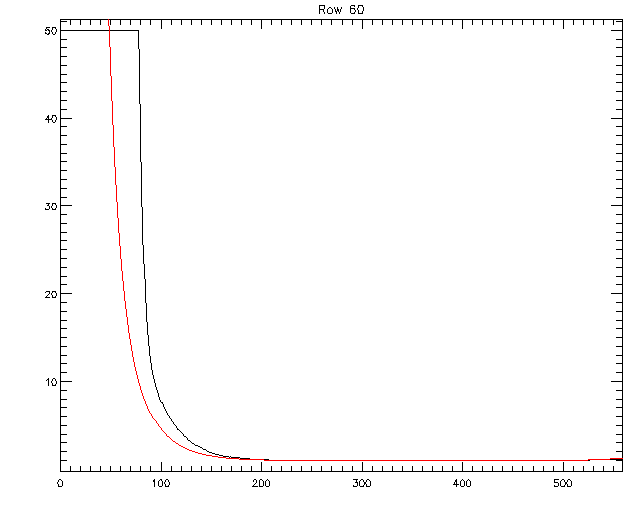11. Plot of ray at row 60, about 30 degrees S of pylon. Black is 1998 values, red is new computed values.12. Plot of ray for row 20, about 10 degrees S of pylon. Black is 1998 values, red is new computed values.13. This is nfrth (Fig. 8) after smoothing and applying a median filter on the lower 200 rows, replacing upper 200 rows with these rows flipped, and using an average of all the rows in between.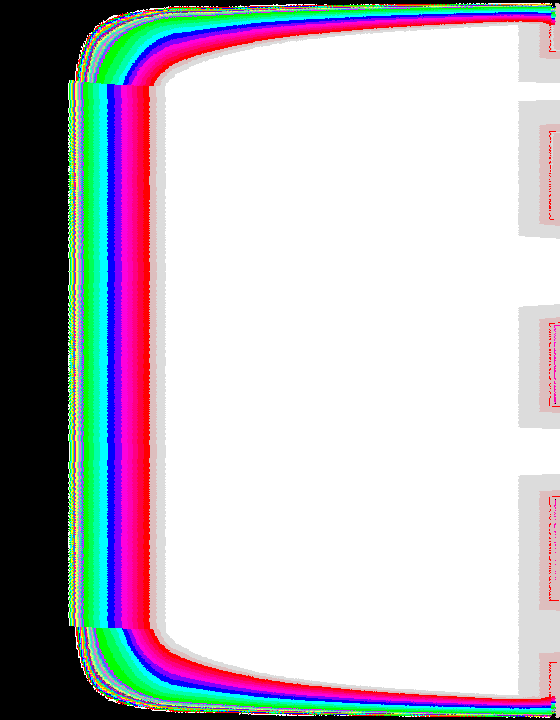14. For comparison, here is the inverse of 1998 vig correction in r-theta. 15. This is my first attempt at the vignetting correction array computed from the rth after smoothing/flipping. Since I didn't like how it looked near the pylon and center, I decided to try smoothing the array in the y (or theta) direction.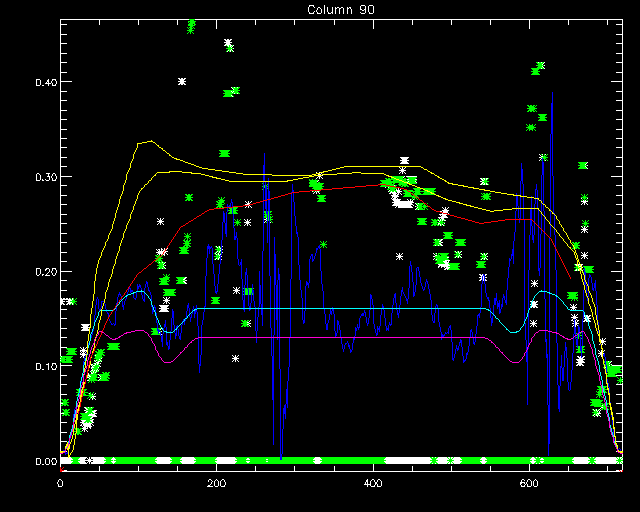16. Here is an example of how I fit/drew a curve in the theta (y) direction. The white stars are values from +/- 1 column and the green stars represent the mean values of stars in a 5x5 box for each pixel in column 90. The dark blue is column 90 from frth0 (Fig. 8). The light blue is column 90 from nfrth (Fig. 13). The magenta line is column 85 from nfrth. The red line is the line I drew for column 90. The yellow lines are the lines drawn for columns 95 and 100.17. nfrth2: This is the resulting array from doing the manual fit to the columns. 18. This is the new vignetting correction array computed from nfrth2 (column fitting applied, Fig. 17). 19. This is the old vignetting correction array from August 1998. 20. This is Fig. 19 (old) minus Fig. 18 (new).